x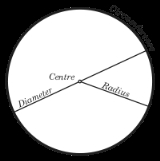Encyclopedia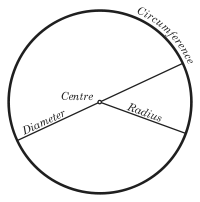In classical geometry
Geometry
Geometry arose as the field of knowledge dealing with spatial relationships. Geometry was one of the two fields of pre-modern mathematics, the other being the study of numbers ....

, a radius of a circle
Circle
A circle is a simple shape of Euclidean geometry consisting of those points in a plane that are a given distance from a given point, the centre. The distance between any of the points and the centre is called the radius....

or sphere
Sphere
A sphere is a perfectly round geometrical object in three-dimensional space, such as the shape of a round ball. Like a circle in two dimensions, a perfect sphere is completely symmetrical around its center, with all points on the surface lying the same distance r from the center point...

is any line segment
Line segment
In geometry, a line segment is a part of a line that is bounded by two end points, and contains every point on the line between its end points. Examples of line segments include the sides of a triangle or square. More generally, when the end points are both vertices of a polygon, the line segment...

from its center
Centre (geometry)
In geometry, the centre of an object is a point in some sense in the middle of the object. If geometry is regarded as the study of isometry groups then the centre is a fixed point of the isometries.-Circles:...

to its perimeter
Perimeter
A perimeter is a path that surrounds an area. The word comes from the Greek peri and meter . The term may be used either for the path or its length - it can be thought of as the length of the outline of a shape. The perimeter of a circular area is called circumference.- Practical uses :Calculating...

. By extension, the radius of a circle or sphere is the length
Length
In geometric measurements, length most commonly refers to the longest dimension of an object.In certain contexts, the term "length" is reserved for a certain dimension of an object along which the length is measured. For example it is possible to cut a length of a wire which is shorter than wire...

of any such segment, which is half the diameter
Diameter
In geometry, a diameter of a circle is any straight line segment that passes through the center of the circle and whose endpoints are on the circle. The diameters are the longest chords of the circle...

. If the object does not have an obvious center, the term may refer to its circumradius, the radius of its circumscribed circle
Circumscribed circle
In geometry, the circumscribed circle or circumcircle of a polygon is a circle which passes through all the vertices of the polygon. The center of this circle is called the circumcenter....

or circumscribed sphere
Circumscribed sphere
In geometry, a circumscribed sphere of a polyhedron is a sphere that contains the polyhedron and touches each of the polyhedron's vertices. The word circumsphere is sometimes used to mean the same thing...

. In either case, the radius may be more than half the diameter, which is usually defined as the maximum distance between any two points of the figure. The inradius of a geometric figure is usually the radius of the largest circle or sphere contained in it. The inner radius of a ring, tube or other hollow object is the radius of its cavity.

For regular polygons, the radius is the same as its circumradius. The inradius of a regular polygon is also called apothem
Apothem
The apothem of a regular polygon is a line segment from the center to the midpoint of one of its sides. Equivalently, it is the line drawn from the center of the polygon that is perpendicular to one of its sides. The word "apothem" can also refer to the length of that line segment. Regular polygons...

. In graph theory
Graph theory
In mathematics and computer science, graph theory is the study of graphs, mathematical structures used to model pairwise relations between objects from a certain collection. A "graph" in this context refers to a collection of vertices or 'nodes' and a collection of edges that connect pairs of...

, the radius of a graph is the minimum over all vertices u of the maximum distance from u to any other vertex of the graph.

The name comes from Latin
Latin
Latin is an Italic language originally spoken in Latium and Ancient Rome. It, along with most European languages, is a descendant of the ancient Proto-Indo-European language. Although it is considered a dead language, a number of scholars and members of the Christian clergy speak it fluently, and...

radius, meaning "ray" but also the spoke of a chariot wheel. The plural of radius is radii.

The radius of the circlest with perimeter
Perimeter
A perimeter is a path that surrounds an area. The word comes from the Greek peri and meter . The term may be used either for the path or its length - it can be thought of as the length of the outline of a shape. The perimeter of a circular area is called circumference.- Practical uses :Calculating...

(circumference
Circumference
The circumference is the distance around a closed curve. Circumference is a special perimeter.-Circumference of a circle:The circumference of a circle is the length around it....

) C is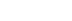The radius of a circle with area
Area
Area is a quantity that expresses the extent of a two-dimensional surface or shape in the plane. Area can be understood as the amount of material with a given thickness that would be necessary to fashion a model of the shape, or the amount of paint necessary to cover the surface with a single coat...

A is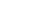To compute the radius of a circle going through three points P1, P2, P3, the following formula can be used: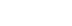where θ is the angle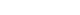This formula uses the Sine Rule.

The radius can be computed from the side s by: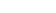whereThe radius of a d-dimensional hypercube with side s is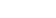The atomic radius of a chemical element is a measure of the size of its atoms, usually the mean or typical distance from the nucleus to the boundary of the surrounding cloud of electrons...

Bend radius, which is measured to the inside curvature, is the minimum radius one can bend a pipe, tube, sheet, cable or hose without kinking it, damaging it, or shortening its life. The smaller the bend radius, the greater is the material flexibility...

The Bohr radius is a physical constant, approximately equal to the most probable distance between the proton and electron in a hydrogen atom in its ground state. It is named after Niels Bohr, due to its role in the Bohr model of an atom...

In Riemannian geometry, the filling radius of a Riemannian manifold X is a metric invariant of X. It was originally introduced in 1983 by Mikhail Gromov, who used it to prove his systolic inequality for essential manifolds, vastly generalizing Loewner's torus inequality and Pu's inequality for the...

in Riemannian geometry
The minimum railway curve radius, the shortest design radius, has an important bearing on constructions costs and operating costs and, in combination with superelevation in the case of train tracks, determines the maximum safe speed of a curve. Superelevation is not a factor on tramway tracks...

The radius is one of the two large bones of the forearm, the other being the ulna. It extends from the lateral side of the elbow to the thumb side of the wrist and runs parallel to the ulna, which exceeds it in length and size. It is a long bone, prism-shaped and slightly curved longitudinally...

Radius of gyration or gyradius is the name of several related measures of the size of an object, a surface, or an ensemble of points. It is calculated as the root mean square distance of the objects' parts from either its center of gravity or an axis....